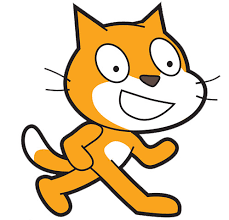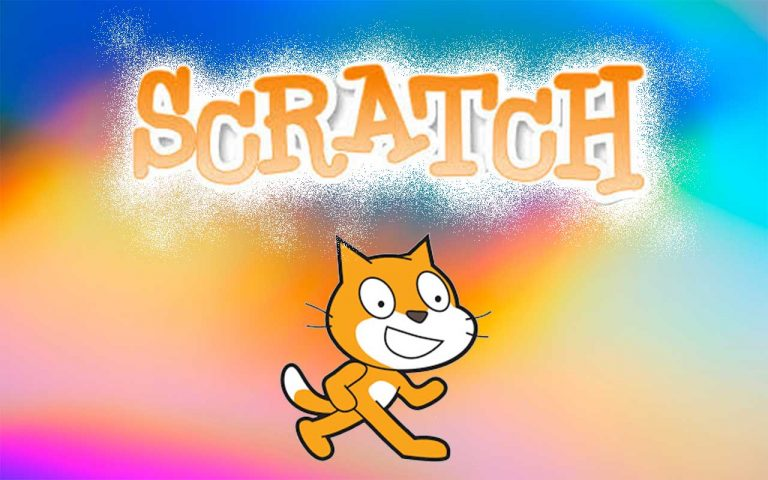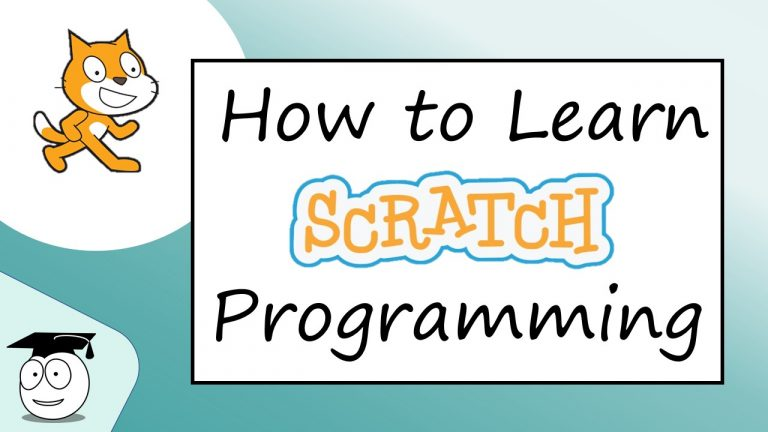# learnscratch

###### simple tutorials for international students

The Scratch programming language is free, fun and easy to learn.

Most programming languages are text-based so a learner has to gain experience writing the code, but Scratch programming is easier as it uses blocks. The learner selects blocks, puts them together and runs the program in a simple way making it the most fun and easiest way to learn programming. We have two approaches detailed below, these are

1. 10 stages of Learning Scratch
2. Famous Tasks to Learn Scratch

### 10 stages of Learning Scratch

Why not learn by doing?  Scratch is easy to learn as you complete simple tasks.

Here we set a series of 10 small tasks, starting from very simple, and increasingly using more tools that help you both learn how to use Scratch, and also how to learn programming concepts.The 10 stages are as follows:

1. shapes
2. game
3. calculator
4. xylophone
5. animation
6. lists
7. maze
8. race
9. functions
10. algorithms

## 10 Stages of Learning Scratch

Complete the set of ten tasks in order to gain knowledge of using Scratch and improve your coding skills and understanding.#### #1 shapes

The first lesson shows how to use Scratch.

We use Scratch to learn about the basic programming concepts.

In this lesson we use several blocks as instructions, these instructions put together make a program.

When we run the program the cat will follow the instructions.#### #2 game

The second lesson mixes some crucial programming concepts with some Scratch based instructions.

We use the programming concept of a conditional statement often called an ‘if’ statement. Both an operator and a loop are also included.

Most of the instructions involve the motion blocks but the paint editor is also used. The looks block includes the ‘say ___ for 2 seconds’ which acts like a print command in text-based programming.

When we run the program the cat will follow the instructions.#### #3 calculator

As you may expect the calculator uses numbers with the operators (+ – * /) and the ‘join’ block.

The numbers are stored in variables which is another important programming concept.

Getting input from a user is not as common in Scratch or in programming but it is used.#### #4 xylophone

The fourth lesson we use Scratch to create a xylophone.

The task is not difficult and does not really include and useful concepts in the program blocks.

But the goal of the lesson is to consider how to complete the task – the easiest way.

Using this logic is important when we design programs. Using logic is a vital component in learning how to code.#### #5 animation

In this lesson we see the use of event programming. This is when an action occurs as a result of an event happening.

We use Scratch to react when two objects touch.

Another feature we see in Scratch is the use of costumes to change the appearance of the sprite object (e.g. make the cat appear to walk)#### #6 lists

This additional lesson introduces lists, often called arrays in programming languages.

We use a loop to iterate through the list – meaning go through each value in a list.

The task requires some programming logic to be completed, either using two loops with an inner loop, or even better an if statement.#### #7 maze

By now you should be able to make a program by yourself. n these instructions you have three options:

• try by yourself
• follow an outline for the design
• use detailed instructions#### #8 race

In this lesson we reuse the code from the last task. Code reuse is an important feature of programming.#### #9 functions

This additional lesson introduces functions. Functions are one of the most important features of programming.

We often need to make a solution to a problem by breaking that problem down into smaller issues.

We code each part using functions. There are other benefits of using functions such as code reuse and ease of reading the code.#### #10 algorithms

The final task id the most difficult using programming techniques to sort numbers into order using a sorting algorithm.

## Famous Tasks to Learn Scratch

The Scratch programming language is free, fun and easy to learn. Most programming languages are text-based so a learner has to gain experience writing the code, but Scratch programming is easier as it uses blocks.

The learner selects blocks, puts them together and runs the program in a simple way making it the most fun and easiest way to learn programming.

#### Start Scratch programming

Scratch in Simple English: An easy to understand tutorial for beginners on how to learn Scratch.

If you want to learn how to use Scratch, this tutorial for beginners is for you. Introduction Scratch Programming | How to Learn Scratch

Scratch in Simple English: An easy to understand tutorial for beginners on how to learn Scratch. How to Learn Scratch Programming | Starter

Using Scratch for programming involves learning what the blocks do, so in this series of videos there are tutorials on how to use the various tools, the different blocks. Each video shows the Scratch programming to complete a simple task.

#### penAn easy to understand tutorial for beginners on how to learn Scratch. In this video we write your name with the pen in Scratch.

#### pongHow to Learn Scratch Programming | Beginners | Pong

Create the pong game where a paddle keeps a ball bouncing around the screen. Part 2 adds extra characters that are animals, sound effects and a score.

#### marksHow to use Scratch variables and learn Scratch operators like addition and multiplication to calculate marks.
Learn Scratch in Simple English: An easy to understand tutorial for beginners on how to learn Scratch.

#### listsHow to Learn Scratch Programming | Loops | Lists

How to use lists and loops in Scratch to loop through a list elements.

In this video we use nested loops to create an ordered list of color items.

Learn Scratch in Simple English: An easy to understand tutorial for beginners on how to learn Scratch.

#### Scratch 3

Scratch programming is the same in Scratch 3 although the interface looks different. In these videos we use the new version and show the differences and also how the use the MyBlock block.

How to Learn Scratch Programming | Scratch 3

How to use Scratch Desktop. Compare the new version Scratch 3.0 with the popular Scratch 2. Learn Scratch in Simple English: An easy to understand tutorial for beginners on how to learn Scratch.

Learn Python Coding from Scratch

Scratch MyBlock is similar to functions in python so here is an example in both scratch and python of using a function.